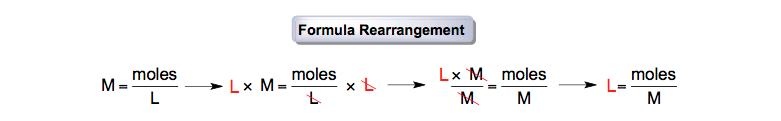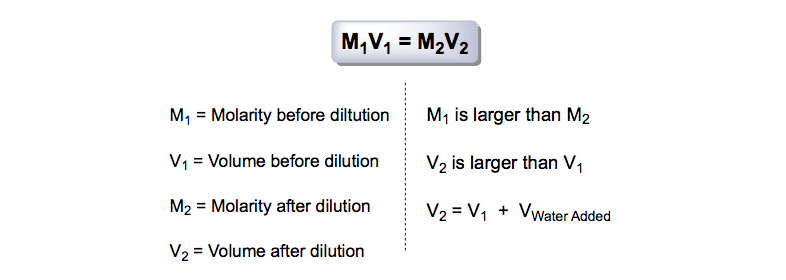Ch.4 - Chemical Quantities & Aqueous ReactionsWorksheetSee all chapters
 Ch.1 - Intro to General Chemistry 2hrs & 53mins 0% complete Worksheet Ch.2 - Atoms & Elements 2hrs & 40mins 0% complete Worksheet Ch.3 - Chemical Reactions 3hrs & 25mins 0% complete Worksheet BONUS: Lab Techniques and Procedures 1hr & 38mins 0% complete Worksheet BONUS: Mathematical Operations and Functions 47mins 0% complete Worksheet Ch.4 - Chemical Quantities & Aqueous Reactions 3hrs & 30mins 0% complete Worksheet Ch.5 - Gases 3hrs & 47mins 0% complete Worksheet Ch.6 - Thermochemistry 2hrs & 28mins 0% complete Worksheet Ch.7 - Quantum Mechanics 2hrs & 35mins 0% complete Worksheet Ch.8 - Periodic Properties of the Elements 1hr & 57mins 0% complete Worksheet Ch.9 - Bonding & Molecular Structure 2hrs & 5mins 0% complete Worksheet Ch.10 - Molecular Shapes & Valence Bond Theory 1hr & 31mins 0% complete Worksheet Ch.11 - Liquids, Solids & Intermolecular Forces 3hrs & 40mins 0% complete Worksheet Ch.12 - Solutions 2hrs & 17mins 0% complete Worksheet Ch.13 - Chemical Kinetics 2hrs & 22mins 0% complete Worksheet Ch.14 - Chemical Equilibrium 2hrs & 26mins 0% complete Worksheet Ch.15 - Acid and Base Equilibrium 4hrs & 42mins 0% complete Worksheet Ch.16 - Aqueous Equilibrium 3hrs & 48mins 0% complete Worksheet Ch. 17 - Chemical Thermodynamics 1hr & 44mins 0% complete Worksheet Ch.18 - Electrochemistry 2hrs & 58mins 0% complete Worksheet Ch.19 - Nuclear Chemistry 1hr & 33mins 0% complete Worksheet Ch.20 - Organic Chemistry 3hrs 0% complete Worksheet Ch.22 - Chemistry of the Nonmetals 2hrs & 1min 0% complete Worksheet Ch.23 - Transition Metals and Coordination Compounds 1hr & 54mins 0% complete Worksheet

# Molarity

See all sectionsJules Bruno

In a solution, the solute is the smaller portion that is being dissolved by the solvent, the larger portion usually in the form of H2O. Molarity is the term used to describe the solubility of the solute within the solvent in the creation of a solution.

Molarity Formula

Molarity serves as the bridge between moles and volume. It is the concentration of a solution represented as moles of solute per liter of solution:Molarity Formula

This would mean a solution that is 0.10 M NaCl:Understanding Molarity

Although moles per liter are the traditional units for molarity, you can also use mg/mL because it is proportionally equivalent.

Molarity Calculations

From the molarity formula, you can calculate volume, grams or the moles of solute.

To calculate molarity from mass (in grams, milligrams, etc.) and volume (milliliters, liters, microliters, etc.) we do the following:

PRACTICE 1: Find the molarity from 25.0 g NaCl in 500 mL solution.

STEP 1: Convert the grams given into moles given.Converting from grams to molesSTEP 2: Convert milliliters into liters.Converting from mL to LSTEP 3: To find the molarity of the solution plug in the moles and liters calculated from Steps 1 and 2.Molarity from mass and volume

Now we must calculate the mass from volume and concentration:

PRACTICE 2: Find the grams of NaCl from 50.0 mL of 0.120 M NaCl.

STEP 1: Rearrange the molarity formula in order to isolate the moles.Molarity Formula RearrangementSTEP 2: Convert the milliliters into liters.Volume Conversion

STEP 3: Multiply liters and molarity together to isolate the moles of the compound.Conversion to moles

STEP 4: Convert the moles into grams.Converting from moles to gramsFinally here's how you calculate the volume from mass and concentration:

PRACTICE 3: Find the volume (in mL) of 0.110 M NaCl from 2.50 moles NaCl.

STEP 1: Rearrange the molarity formula in order to isolate the liters.Isolating Liters

STEP 2: Divide moles by molarity to isolate the liters of the compound.Molarity Formula and Liters

STEP 3: Convert liters into millimeters.Conversion of L into mL

Osmolarity

Ionic molarity or osmolarity represents the molarity of dissolved ions in a solution. For example, if you were asked how to calculate the osmolarity of 0.200 M KNO3

STEP 1: Break the ionic compound into its ions.Dissolution of KNO3

STEP 2: Now calculate the osmolarity of the solution with its equation.Osmolarity

Dilutions

Another common idea related to molarity deals with the dilution of stock solutions. In a dilution a concentrated solution is made more diluted by adding water directly to it.Dilution Equation

After Molarity

Eventually you will do calculations that connect molarity to problems with density, molarity vs molality, mole fraction, mass fraction or percent

Beyond these calculations that deal directly with the molarity formula you can bring in stoichiometry. This brings in more complex problems dealing with solution chemistry, acid base titration, pH and pOHJules Bruno

Jules felt a void in his life after his English degree from Duke, so he started tutoring in 2007 and got a B.S. in Chemistry from FIU. He’s exceptionally skilled at making concepts dead simple and helping students in covalent bonds of knowledge.